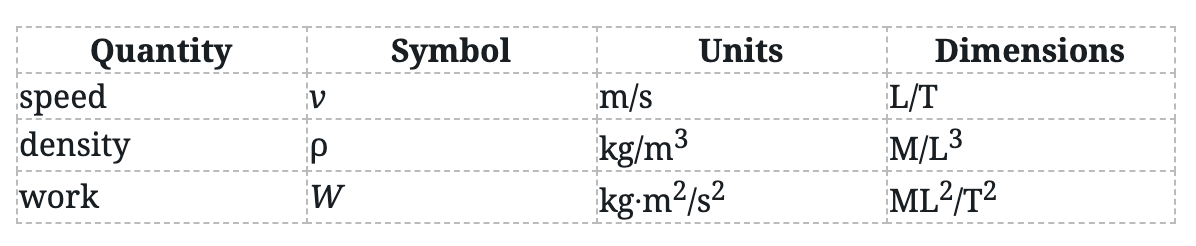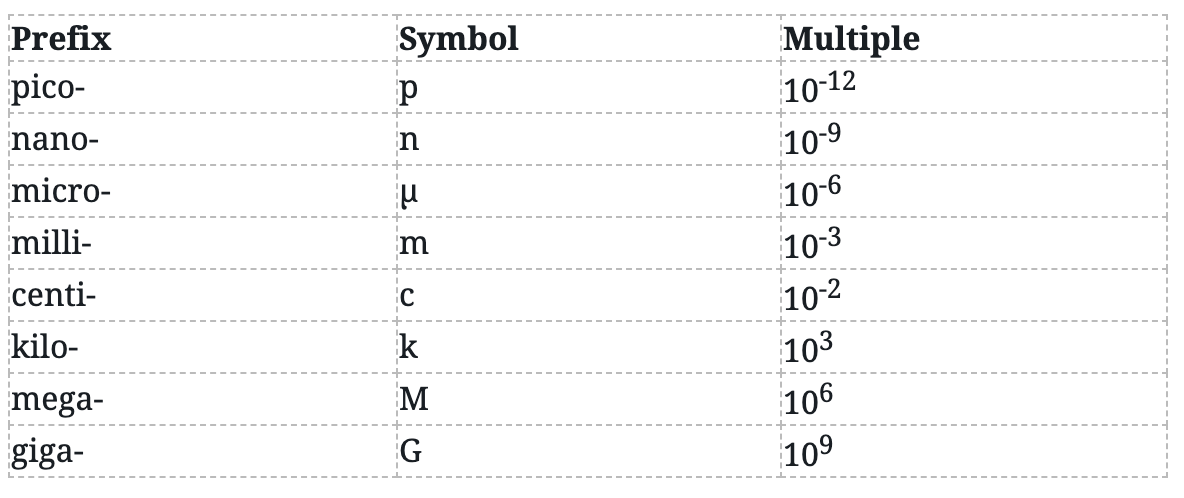Cyber Sale - Only 3 Days Remaining: ALL MCAT Products & Admissions Services

MCAT Content / Translational Motion / Units And Dimensions

### Units and Dimensions

Topic: Translational Motion

Scientists (and the MCAT) use the International System of Units (SI) to express the measurements of physical quantities.

Scientists (and the MCAT) use the Système International d’Unités (SI), also referred to as the International System of Units or the metric system, to express the measurements of physical quantities. The base units of the SI are listed below:Each dimension is an abbreviation for the quantity that is being measured- it does not depend on the particular unit being used.

Any physical quantity can be written in terms of the SI base units. Some examples include:Multiples of the base units that are powers of ten are often abbreviated and precede the symbol for the units. Some of the most common power-of-ten prefixes are:You should memorize this list!

On the MCAT, it won’t be necessary to convert between the American system of units and the metric system. However, you will need to be able to convert with the metric system using the powers-of-ten prefixes.

Key Points

• Scientists (and the MCAT) use the International System of Units (SI) to express the measurements of physical quantities.

• The SI is also referred to as the mks system (m for meters, k for kilograms, and s for seconds).

• Each dimension is an abbreviation for the quantity that is being measured.

• Any physical quantity can be written in terms of the SI base units.

• You will need to be able to convert within the metric system using the powers-of-ten prefixes.

Key Terms

Système International d’Unités (SI), International System of Units, Metric System: The decimal measuring system based on the meter, liter, and gram as units of length, capacity, and weight or mass.

Base units: A fundamental unit that is defined arbitrarily and not by combinations of other units. The base units of the SI system are the meter, kilogram, second, ampere, kelvin, mole, and candela.

Power-of-ten prefixes: The prefixes of the metric system, such as kilo and milli, represent multiplication by powers of ten.

Billing Information